# Lab 9Mar 06 2019

Goals:

• Lasso and ridge regression
• Sensitivity of model selection to transformations
library(tidyverse)

# Lasso and ridge regression

Work through code from Faraway 11.3 and 11.4

Meat fat content example from model selection examples in class:

data(meatspec, package = "faraway")
# Faraway takes the first 172 obs as training
# you shouldn't generally do that without careful
# thought
ind <- 1:172
trainmeat <- meatspec[ind, ]
testmeat <- meatspec[-ind, ]

# center all columns of x for ridge and lasso
x <- scale(meatspec[, -101], center = T, scale = F)
y <- meatspec[, 101]

testx <- x[-ind, ]
testy <- y[-ind]
trainx <- x[ind, ]
trainy <- y[ind]
rmse <- function(x,y) sqrt(mean((x-y)^2))
fit_all <- lm(fat ~ ., data = trainmeat)
(rmse_all <- rmse(testmeat$fat, predict(fit_all, newdata = testmeat))) ##  3.814 Recall that a model with all predictors, had an estimated root mean square prediction error of about 3.81. fit_best <- lm(fat ~ V1+V17+V19+V20+V21+V24+V31+V35+V37+V39+V40+V41+V44+V47+V48+ V51+V53+V57, data = trainmeat) (rmse_best <- rmse(testmeat$fat, predict(fit_best, newdata = testmeat)))
##  1.837097

We also found some smaller models on Monday with smaller error, e.g., 1.84.

## Ridge regression

Estimating the coefficients for a sequence of tuning parameters:

library(MASS)
rgmod <- lm.ridge(I(trainy - mean(trainy)) ~ trainx,
lambda = seq(0, 5e-8, 1e-9))

matplot(rgmod$lambda, coef(rgmod), type = "l", xlab = expression(lambda), ylab = expression(hat(beta)), col = 1)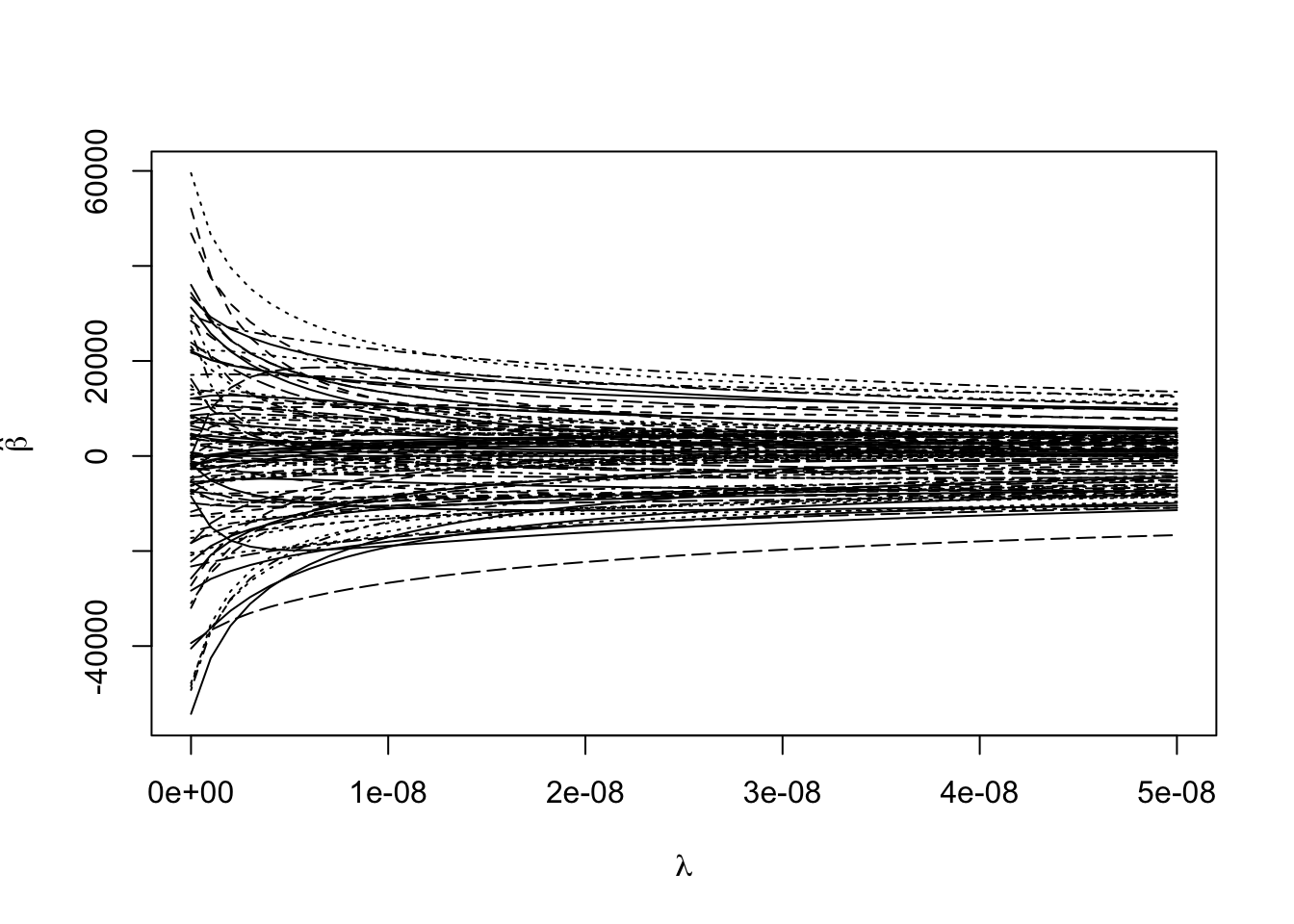Choosing one tuning parameter: (min_gcv <- which.min(rgmod$GCV))
## 1.8e-08
##      19
matplot(rgmod$lambda, coef(rgmod), type = "l", xlab = expression(lambda), ylab = expression(hat(beta)), col = 1) abline(v = rgmod$lambda[min_gcv])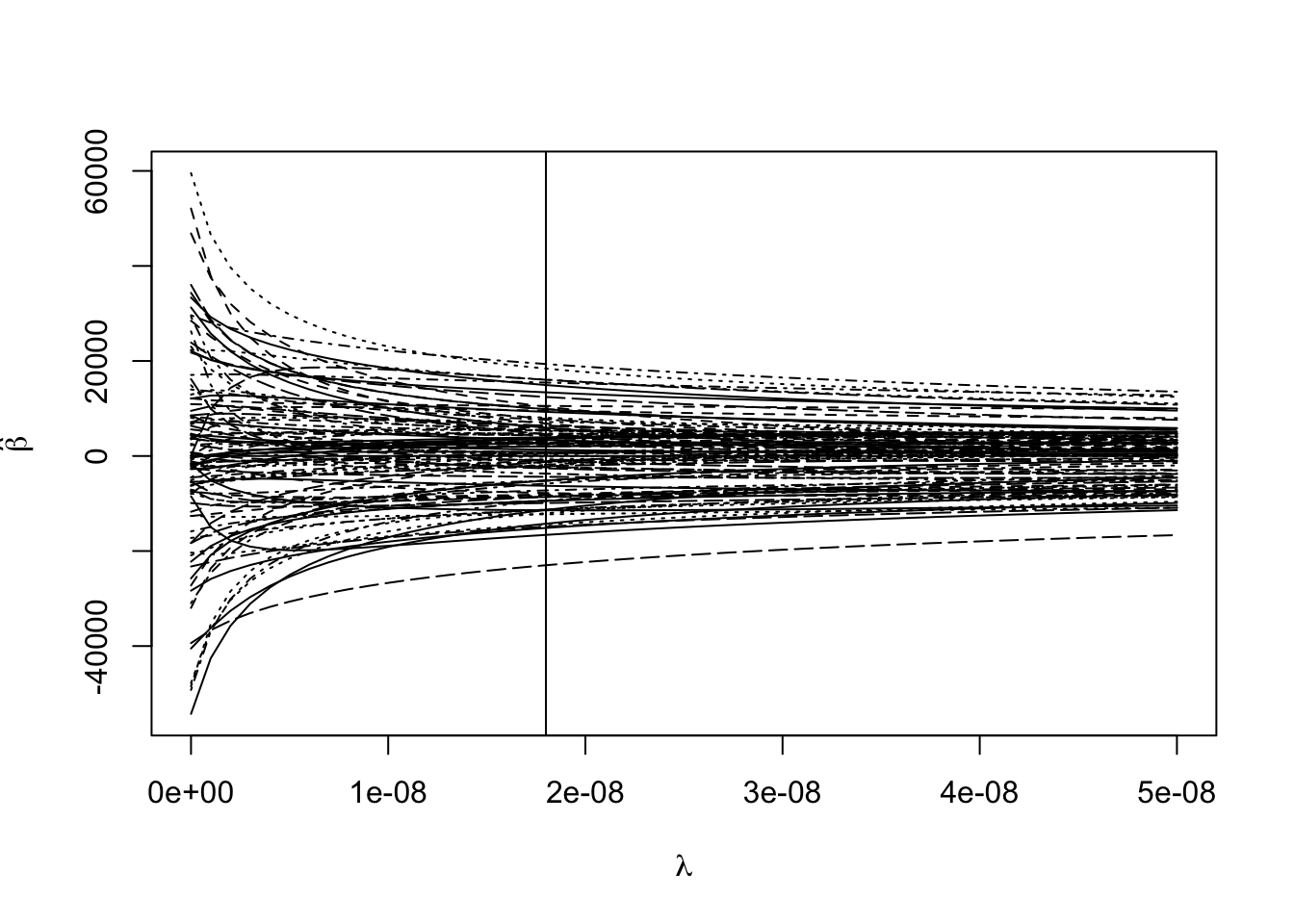Evaluating the predictive accuracy:

ypred <- scale(trainx,
center = F,
scale = rgmod$scales) %*% # scale covariates rgmod$coef[, min_gcv] + # multiply by estimated coefficients
mean(trainy) # add back mean (since response was centered)

rmse(ypred, trainy)
##  0.8159824
ypred <- scale(testx,
center = F,
scale = rgmod$scales) %*% # scale covariates rgmod$coef[, min_gcv] + # multiply by estimated coefficients
mean(trainy) # add back mean (since response was centered)
rmse(ypred, testy)
##  4.067914

One particularly bad prediction (looks OK to me, why does Faraway get something different?:

c(ypred, testmeat$fat) ##  11.32387 34.80000 rmse(ypred[-13], testmeat$fat[-13])
##  1.954433
cbind(ypred, testy)
##               testy
## 173 48.047247  47.7
## 174 23.143626  25.5
## 175  8.944386  10.6
## 176  3.461404   2.0
## 177  6.141724   4.7
## 178  6.741146   6.8
## 179 10.116475   8.6
## 180 11.781558  11.2
## 181 13.553112  13.8
## 182 14.937346  17.7
## 183 28.464430  23.3
## 184 24.774611  29.0
## 185 11.323870  34.8
## 186 46.180519  47.8
## 187  9.960860  11.1
## 188  4.791950   2.9
## 189  9.148401   4.8
## 190  5.900053   5.6
## 191  6.465477   6.2
## 192  6.794227   6.4
## 193  6.004676   6.8
## 194  6.815092   7.1
## 195  7.820161   7.3
## 196  8.016253   7.9
## 197 10.328492   9.2
## 198  9.358988  10.1
## 199 11.897665  10.7
## 200  7.628819  11.2
## 201 11.506780  12.5
## 202 14.621129  14.3
## 203 11.126646  16.0
## 204 16.681274  17.0
## 205 16.686706  18.1
## 206 20.448977  19.4
## 207 23.345195  24.8
## 208 26.978289  27.2
## 209 29.363296  28.4
## 210 29.227825  29.2
## 211 29.045001  31.3
## 212 31.692496  33.8
## 213 37.691766  35.5
## 214 41.439211  42.5
## 215 46.180519  47.8
which.max(abs(ypred - testy))
##  13
rmse(ypred[-13], testy[-13])
##  1.954433

## Lasso

library(lars)

Example with a smaller set of predictors (the state life expectancy data from last week)

Estimating the coefficients for a sequence of tuning parameters:

statedata <- data.frame(state.x77, row.names = state.abb)
lmod <- lars(as.matrix(statedata[, -4]), statedata$Life) Choosing one tuning parameter: plot(lmod)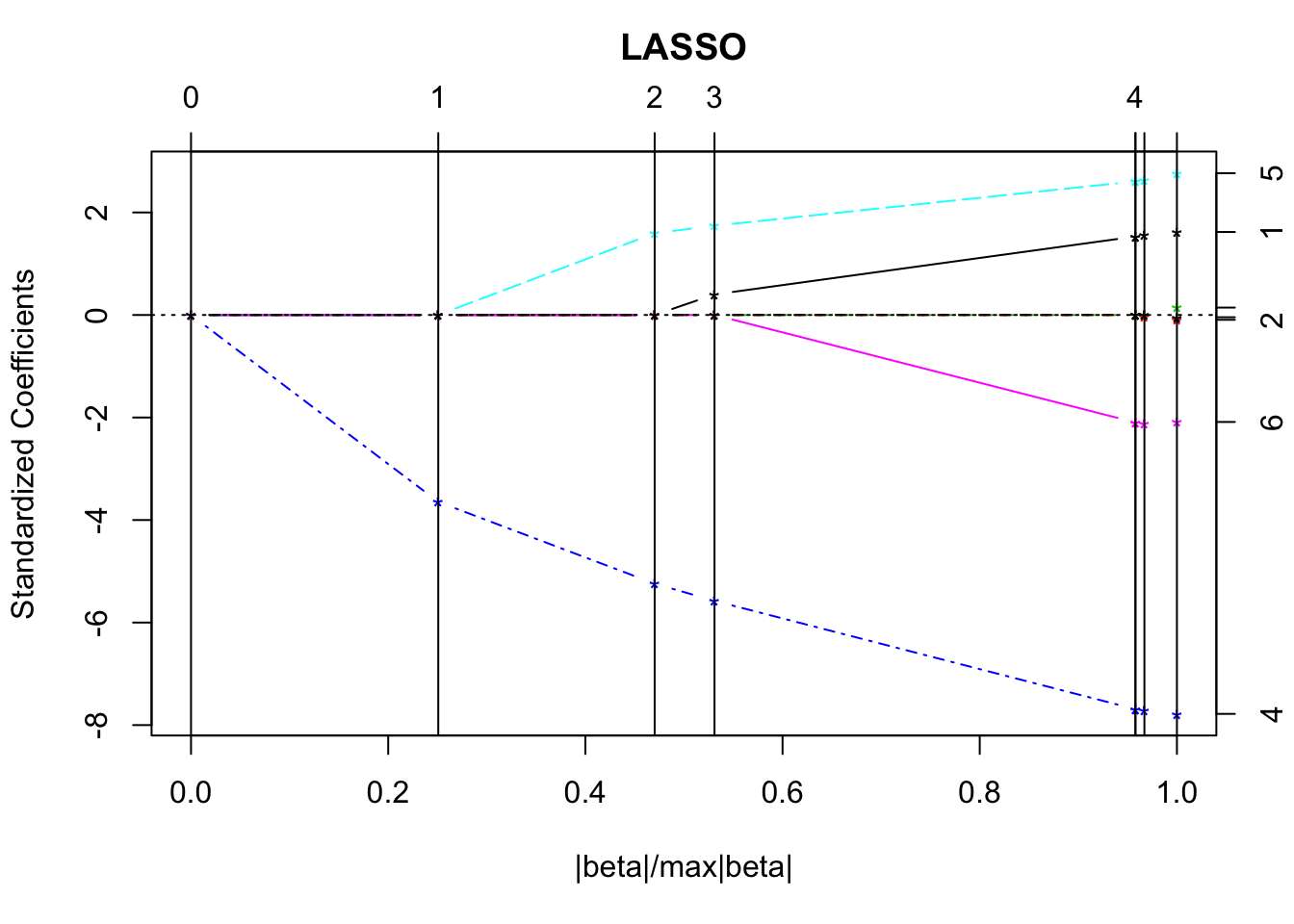set.seed(123) cvlmod <- cv.lars(as.matrix(statedata[, -4]), statedata$Life)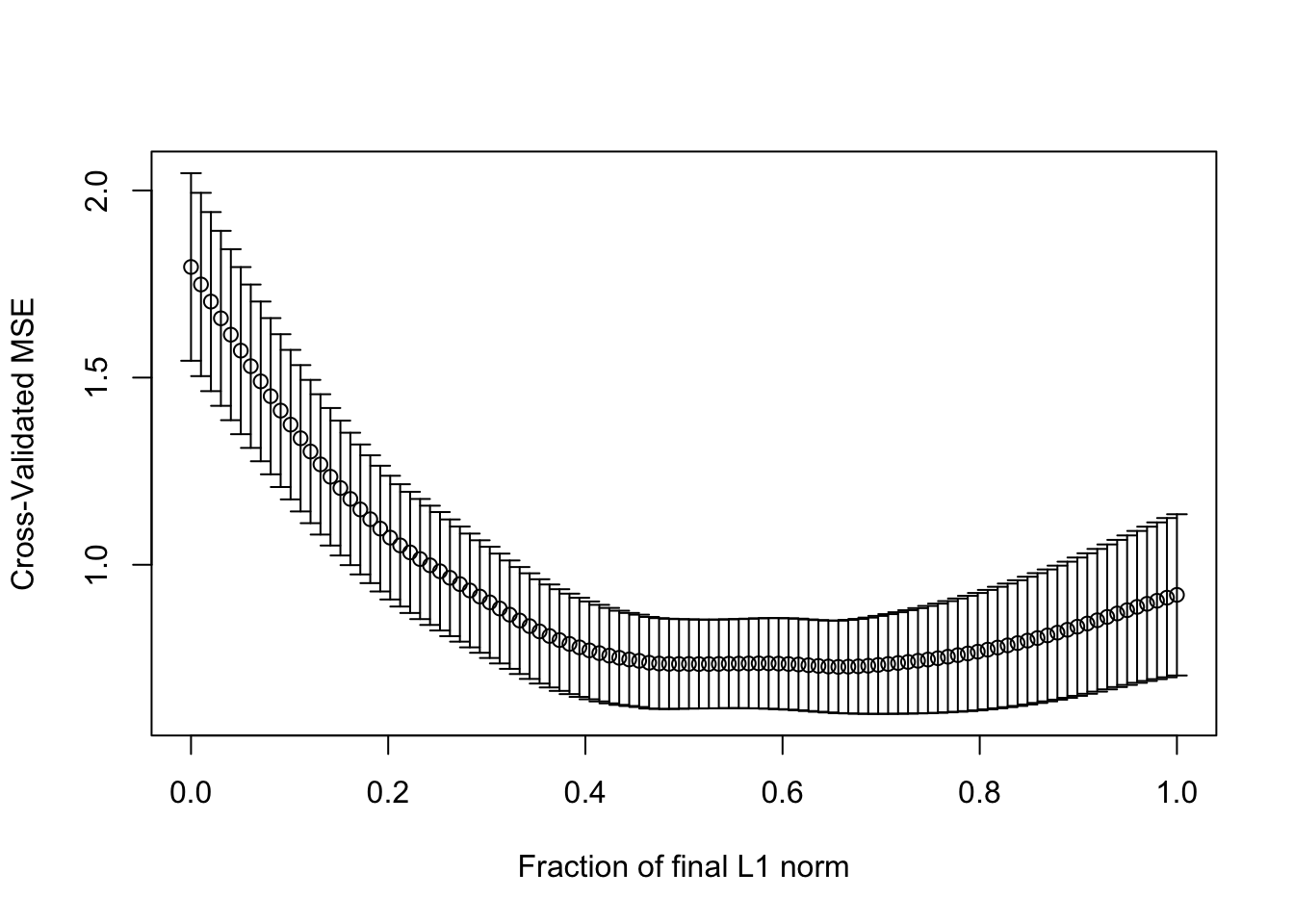(s <- cvlmod$index[which.min(cvlmod$cv)])
##  0.6565657

Compare the coefficients in the lasso model:

predict(lmod, s = s, type = "coef", mode = "fraction")$coef ## Population Income Illiteracy Murder HS.Grad ## 2.345262e-05 0.000000e+00 0.000000e+00 -2.398786e-01 3.528871e-02 ## Frost Area ## -1.694932e-03 0.000000e+00 to those from the least squares fit: coef(lm(Life.Exp ~ Population + Murder + HS.Grad + Frost, statedata)) ## (Intercept) Population Murder HS.Grad Frost ## 7.102713e+01 5.013998e-05 -3.001488e-01 4.658225e-02 -5.943290e-03 Back to the meat fat example Estimating the coefficients for a sequence of tuning parameters: lassomod <- lars(trainx, trainy) Choosing one tuning parameter: set.seed(123) cvout <- cv.lars(trainx, trainy)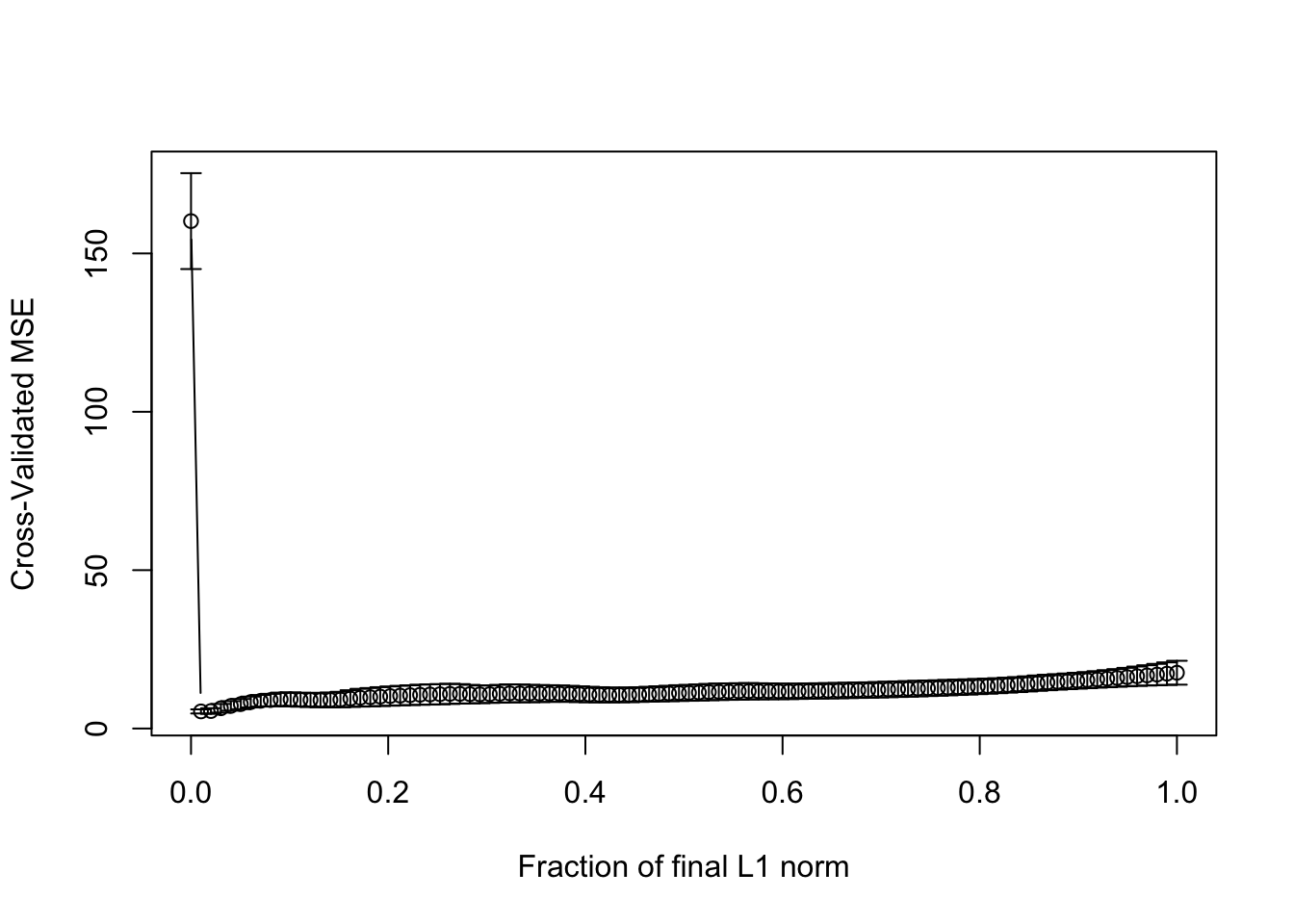(best_s <- cvout$index[which.min(cvout$cv)]) ##  0.01010101 Evaluating the predictive accuracy: predlars <- predict(lassomod, testx, s = best_s, mode = "fraction") rmse(testy, predlars$fit)
##  2.132218

Examing which coefficients are non-zero:

predlars <- predict(lassomod, s = best_s, type = "coef", mode = "fraction")
plot(predlars$coef, type = "h", ylab = "Coefficient")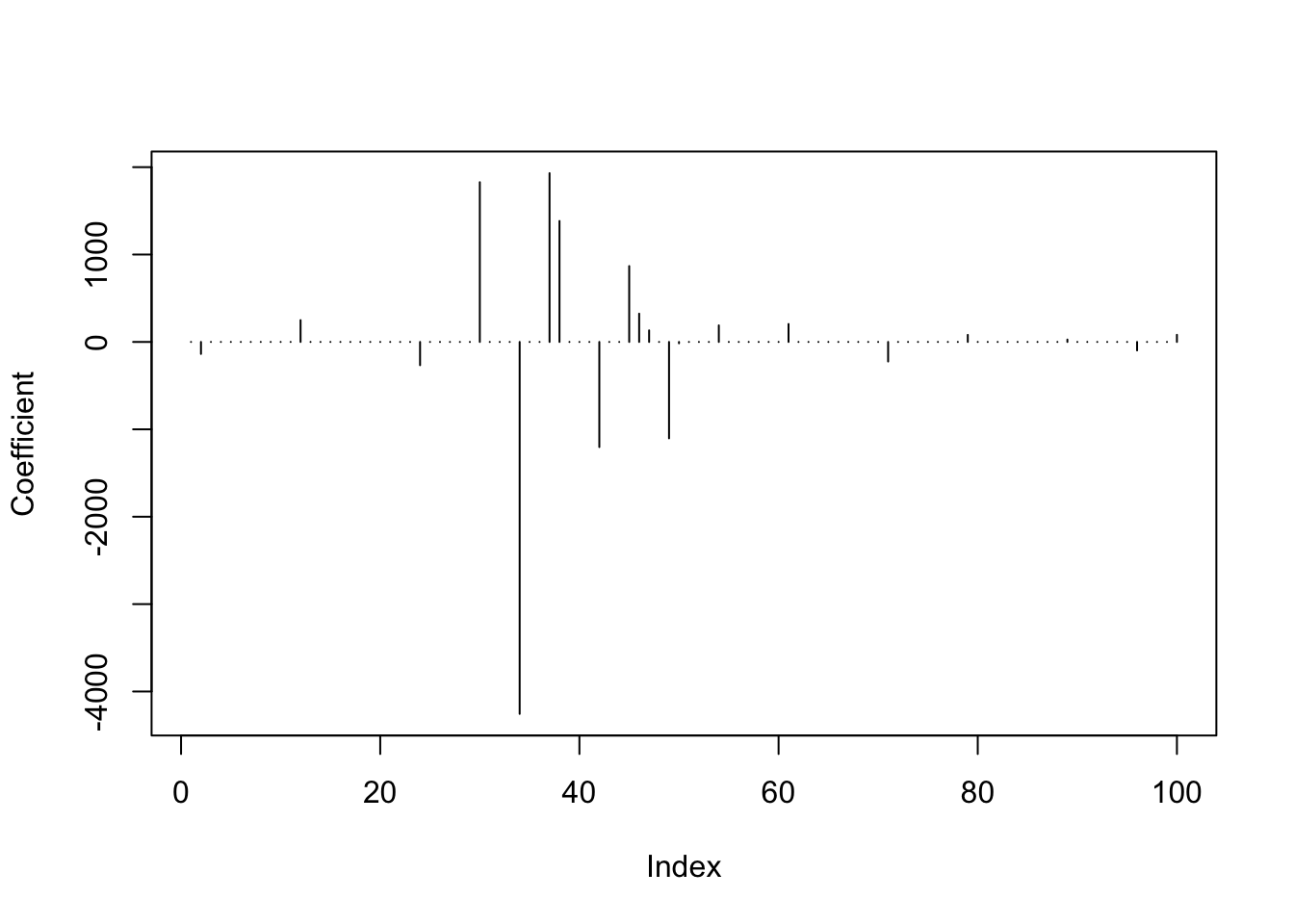sum(predlars$coef != 0)
##  20

# Sensitivity of model selection

Work through code in Faraway 10.3

library(leaps)
source("http://stat552.cwick.co.nz/lecture/fortify-leaps.R")

In class we used regsubsets() to select variables to predict life expectancy from other state level data. In particular, imagine we use an exhaustive search and choose a model with the highest adjusted R-squared:

b <- regsubsets(Life.Exp ~ ., data = statedata)
rs <- summary(b)
rs$which[which.max(rs$adjr2),]
## (Intercept)  Population      Income  Illiteracy      Murder     HS.Grad
##        TRUE        TRUE       FALSE       FALSE        TRUE        TRUE
##       Frost        Area
##        TRUE       FALSE

It’s possible this choice is sensitive both to indivudal points and transformations.

lmod <- lm(Life.Exp ~ ., data = statedata)
broom::augment(lmod) %>%
arrange(desc(.hat))
## # A tibble: 50 x 16
##    .rownames Life.Exp Population Income Illiteracy Murder HS.Grad Frost
##    <chr>        <dbl>      <dbl>  <dbl>      <dbl>  <dbl>   <dbl> <dbl>
##  1 AK            69.3        365   6315        1.5   11.3    66.7   152
##  2 CA            71.7      21198   5114        1.1   10.3    62.6    20
##  3 HI            73.6        868   4963        1.9    6.2    61.9     0
##  4 NV            69.0        590   5149        0.5   11.5    65.2   188
##  5 NM            70.3       1144   3601        2.2    9.7    55.2   120
##  6 TX            70.9      12237   4188        2.2   12.2    47.4    35
##  7 NY            70.6      18076   4903        1.4   10.9    52.7    82
##  8 WA            71.7       3559   4864        0.6    4.3    63.5    32
##  9 OR            72.1       2284   4660        0.6    4.2    60      44
## 10 ND            72.8        637   5087        0.8    1.4    50.3   186
## # … with 40 more rows, and 8 more variables: Area <dbl>, .fitted <dbl>,
## #   .se.fit <dbl>, .resid <dbl>, .hat <dbl>, .sigma <dbl>, .cooksd <dbl>,
## #   .std.resid <dbl>

Without it, the model selected changes:

b <- regsubsets(Life.Exp ~ ., data = statedata, subset = (state.abb != "AK"))
rs <- summary(b)
rs$which[which.max(rs$adjr2),]
## (Intercept)  Population      Income  Illiteracy      Murder     HS.Grad
##        TRUE        TRUE       FALSE       FALSE        TRUE        TRUE
##       Frost        Area
##        TRUE        TRUE

Notice Area and Population might benefit from a transform:

stripchart(data.frame(scale(statedata)), method = "jitter", las = 2, vertical = TRUE)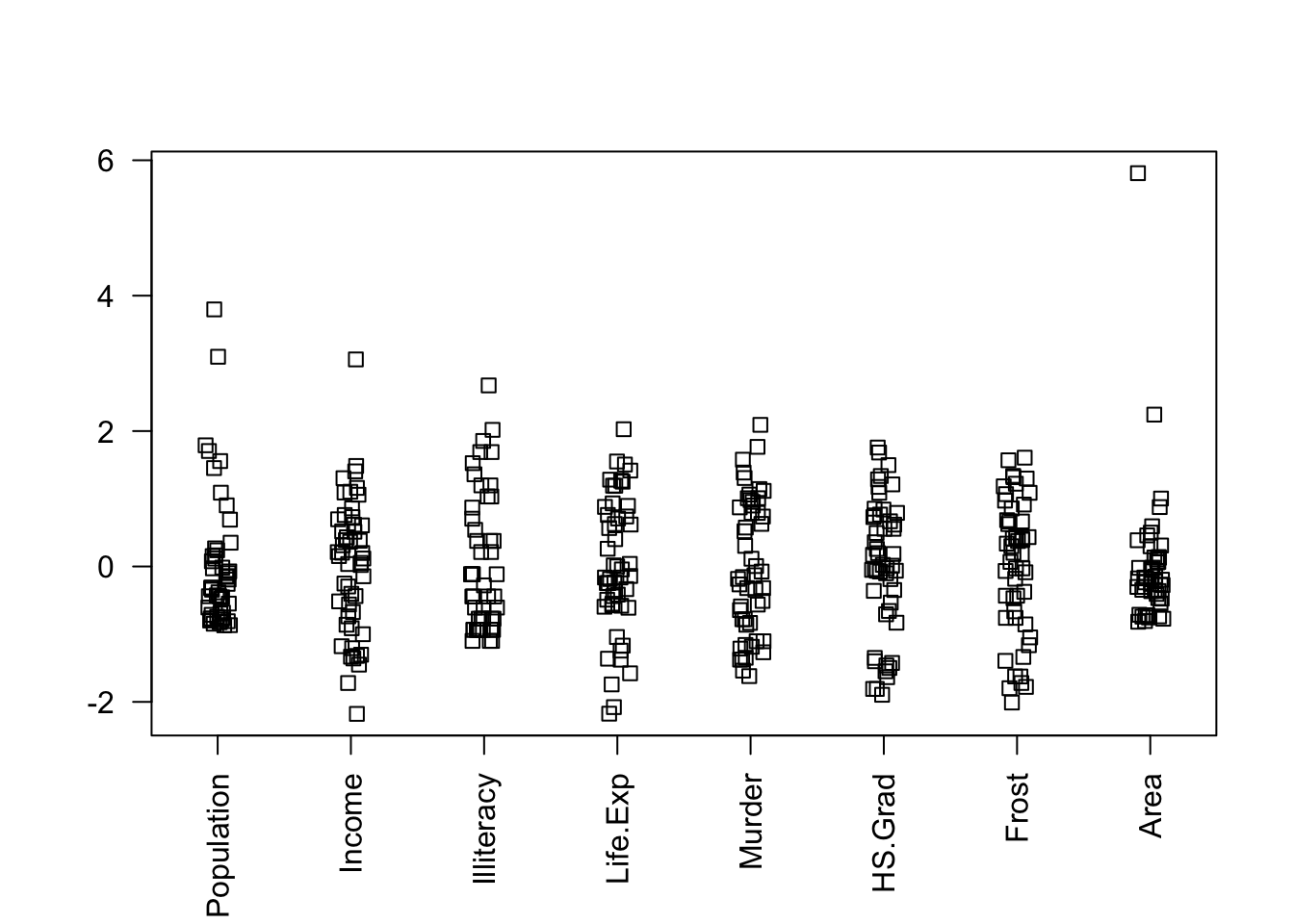If we transform them, the selected model changes:

b <- regsubsets(Life.Exp ~ log(Population) + Income + Illiteracy + Murder + HS.Grad + Frost + log(Area), statedata)
rs <- summary(b)
rs$which[which.max(rs$adjr), ]
##     (Intercept) log(Population)          Income      Illiteracy
##            TRUE            TRUE           FALSE           FALSE
##            TRUE            TRUE            TRUE           FALSE